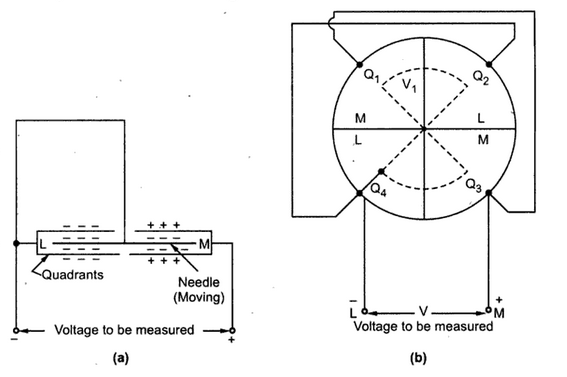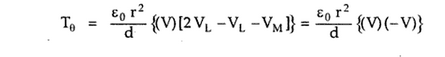### Types of Electrostatic Voltmeters part 2

1.3 Idiostatic connection
This is connection generally used in commercial instruments. In this type of connection, needle is connected to any one of the pairs of quadrant as shown in the Fig 1, directly without external voltage.Fig. 1 Idiostatic connection

The moving needle is negatively charged, the left and quadrant is negatively charged and the right and quadrant is positively charged. The force of attraction on needle due to top and bottom parts of right hand quadrant cancel each other. So there is no motion of needle due to top and bottom parts of left hand quadrant also cancel each other.
Thus the right hand positively charged quadrant attracts the part of the needle near to left hand quadrant while the left hand negatively charged quadrant repels the part of the needle to right and quadrant. This rotates the needle and hence the pointer.
1.3.1Teory of Idiostatic Connection
For idiostatic connection, te external voltage applied to the needle V1 = 0 V. And the potential of quadrant is nothing but the voltage V1 which is applied to the needle directly.
VL        ..        V1   =
While the voltage to be measured is V = VM    VL   .
Thus using these values in the expression of Tθ ,The negative sign is neglected as it indicates the direction of rotation opposite to that which has been assumed.

Note : As torque is proportional to square of the applied voltage, the scale is nonuniform for idiostatic connection. Note that as deflecting torque is proportional to the square of the voltage to be measured, the idiostatic connection is used for a.c. measurement.

1.4 Kelvin Multicellular Voltmeter
It is one of the most important commercial from an electric voltmeter. It is basically a quadrant electrometer with large number of needles and only one quadrant. Basically it is used for a voltage range of 100 to 1000 volts. By modifying basic voltmeter it is possible to measure voltages of the range of 40 V only.
Thus to obtain a very high force for very small voltages, a large number of cells are used in te instruments hence it is called multicellular. The Kelvin multicellular voltmeter is as shown in the Fig 2.Fig. 2 Kelvin Multicellular Voltmeter

The moving mechanism is suspended with the help of Phosphor-Bronze suspension. To protect the suspension against the vibration, the coach spring is used. For the zero adjustment, warm wheel actuated torsion head is used. The pointer and scale are of edgewise type alongwith oil immersed damping vane.
It is very essential to use a safely collar above the pointer to avoid movements of the system caused due to short circuit of quadrants. Two guard plates are fitted inside inside the case of instrument. These are electrically connected to the moving mechanism and metal case of the instrument.
In such instruments, number of cells used increases as the voltage to be measured decreases. For example to measure voltage of the order of 150 V, 10 to 15 cells are required while for the measurement of 3000 V one cell may be sufficient.
The deflection torque for n cells is given by